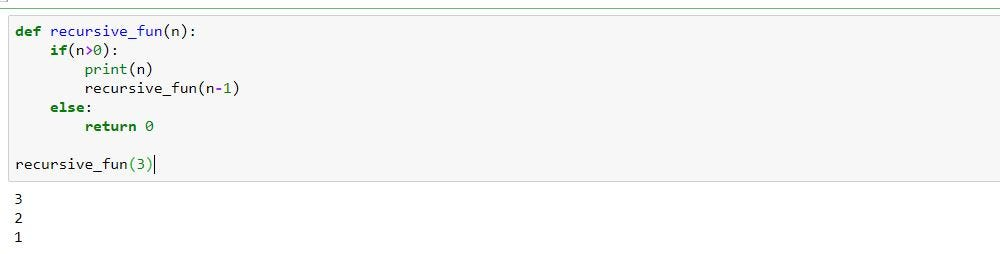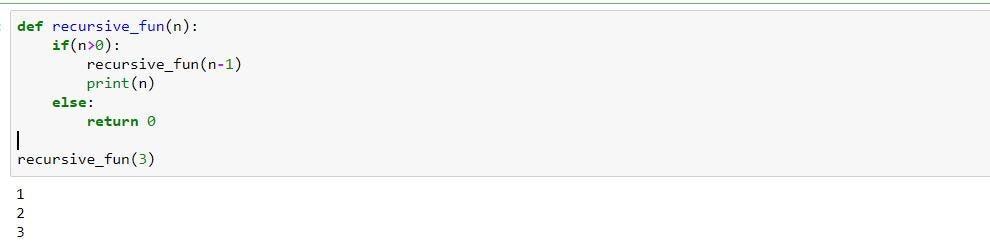1634648400

# Dijkstra was correct: recursion should be simple

Consider how the original problem may be expressed as a smaller problem with some additional operations before you begin working on a solution. The most difficult element of solving a recursive problem is identifying the simpler problem. For some simple issues, such as the ones we used in the examples above, this may be basic, but identifying a pattern needs skills for many more difficult problems.

## Buddha Community1603537200

## While You Don't Understand Recursion, Read Recursion: by Randy Taylor

Recursion is the one idea I constantly use while I solve coding problems. Most of the time I don’t start by thinking “RECURSION WILL SOLVE THIS!”. However recursion just ends up being the logical way to reach an answer. In my professional opinion recursion is the purest form of coding; write a function that will call itself until you get what you want! To implement recursion we will create a helper algorithm. 1) Identify what the smallest input is. 2) Continually break down a larger input into smaller inputs and pass those smaller inputs back into itself until you get the desired answer. 3) Define a “base case” that will stop the Recursion should the answer not be found.

Let’s look at the idea of Recursion first. We are writing code that will execute itself until we get a desired answer or reach a base case. Essentially we are creating a loop. I will illustrate this with pseudo code:

``````for (let recursion =()=>{ …answer? answer = true : false} ; answer === false; recursion())
``````

Much like a traditional for loop the above pseudo code will continue while the second condition is true; the recursion will continue until answer === true. At this point the second statement of the for loop is false terminating the loop. Above if answer === false recursion will call itself again. This is the general idea of recursion. This is why creating a base case is essential to prevent an infinite loop. The “answer” we are looking for might not be present causing recursion to run until the sun burns out.

#algorithms #javascript #recursion #tutorial-for-beginners #iteration #recursion-explained #what-is-recursion #programming1599158640

Anybody reading data structures and algorithm would have come across a topic called “Recursion”. Now here is the best way to understand recursion. Read this ’n’ times until your understanding becomes** “True”**. Haha!! now that’s how simple the recursion is.

Well recursion can be implemented in any language and it has the same outcome. Here i’ll be demonstrating few topics and key concepts on recursion in python🐍. Yes, you read it right one love for python❤.

## Introduction:

A function calling itself until a condition is set true or false is called recursive function. The below image shows to a simple function called “recursive_fun” calling itself ’n’ times.a simple example of recursion

The output of a recursion can be understood by a concept called as tracing which is done to trace the output by a recursive function.

a tracing tree of recursive_fun(forward phase)

the above tracing tree explains a lot about how the repetitive function call takes place to obtain the desired output. Here the recursive_fun is called 3+1 times where for all the 3 times it prints ’n’ but for the last call it fails to satisfy the condition and returns 0. Hence, in any recursion the function call is made n+1 times. Also, on carefully observing the tracing tree we see that the recursive_fun executes print statement before calling itself, this is known as forward phase. A backward phase of recursion is when a function calls itself before executing any statements, i.e all the other statements are executed until there is no further function calls.#recursive #algorithms #python #recursion #data-structures1622168113

## Recursion And Iteration In Python Programming

Acomputer program consists of line-by-line instructions. The computer performs those instructions line-by-line. However, some instructions may be repetitive with a common pattern. Recursion or iteration helps one to write a few lines of codes to perform such repetitive tasks. Suppose a Python list with five-string elements. We wish to print the elements one in a line. This operation needs five lines of codes.

`````` flowers = ['lily', 'tulip', 'rose', 'lavender', 'dandelion']
print(flowers)
print(flowers)
print(flowers)
print(flowers)
print(flowers)
``````

Output:It can be observed that the five lines of codes follow the same pattern. The only difference in each line is the index of the list elements. What if this list contains 100 or 1000 elements? Coding will become a tedious task. These kinds of problems are resolved through either iteration or recursion. Here, the iterative form of the above codes is as follows.

`````` for flower in flowers:
print(flower)
``````

Output:These two lines are sufficient to achieve the task even if the list contains a million elements!

#developers corner #backtracking #dynamic programming #factorial #iteration #iterative #python #python programming #quicksort #recursion #recursive #sorting1592751160

## Correct Your Sitting Posture - How to Sit at Your Desk

#how #correct your sitting posture #how to sit at your desk correctly #how to sit at your desk correctly1625631000

## What's Recursion and Why use it? Beyond Code Live-X 090

(Previously 002) In the words of Woody: “That’s not Recursion! It’s looping with style!”

Follow Beyond Code:(Learn to Code in 15 Minutes a Day)